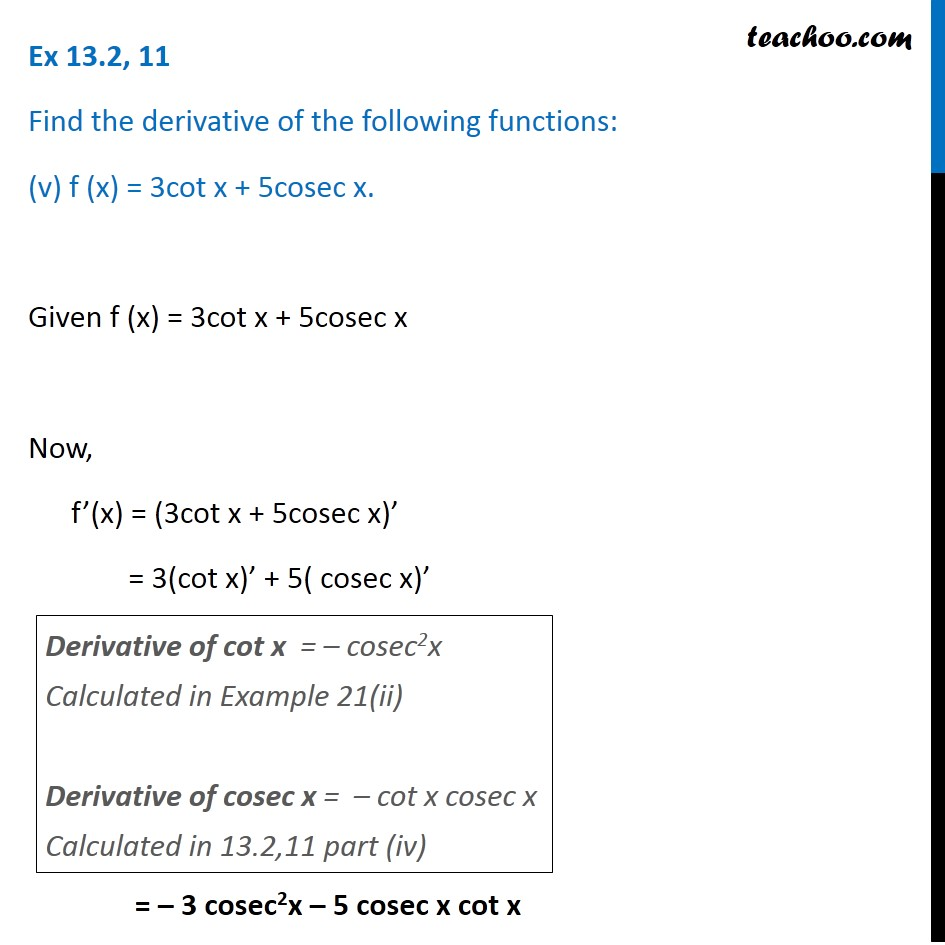Ex 12.2

Chapter 12 Class 11 Limits and Derivatives
Serial order wiseLearn in your speed, with individual attention - Teachoo Maths 1-on-1 Class

### Transcript

Ex 12.2, 11 Find the derivative of the following functions: (v) f (x) = 3cot x + 5cosec x. Given f (x) = 3cot x + 5cosec x Now, f’(x) = (3cot x + 5cosec x)’ = 3(cot x)’ + 5( cosec x)’ = – 3 cosec2x – 5 cosec x cot x Derivative of cot x = – cosec2x Calculated in Example 21(ii) Derivative of cosec x = – cot x cosec x Calculated in 13.2,11 part (iv)